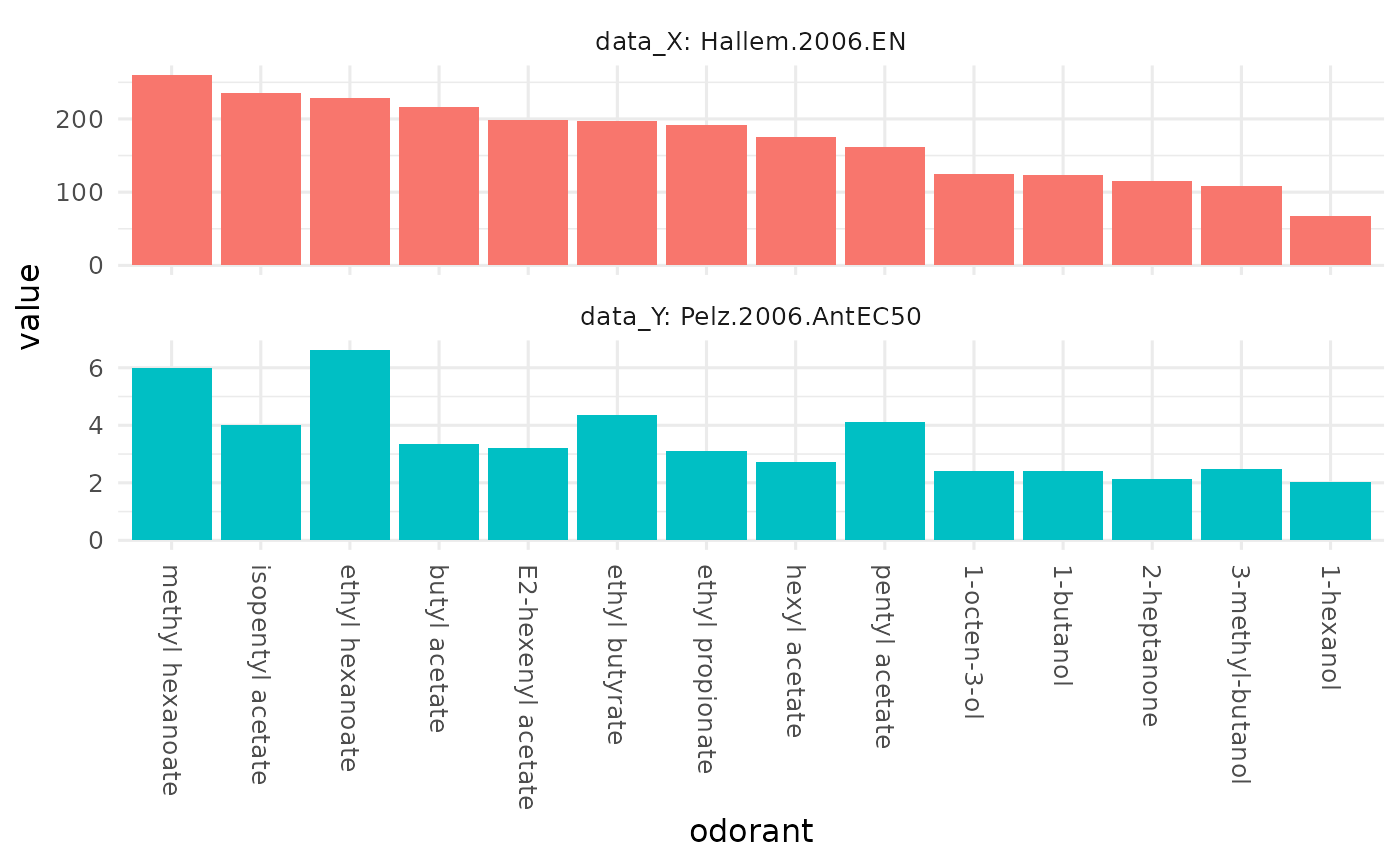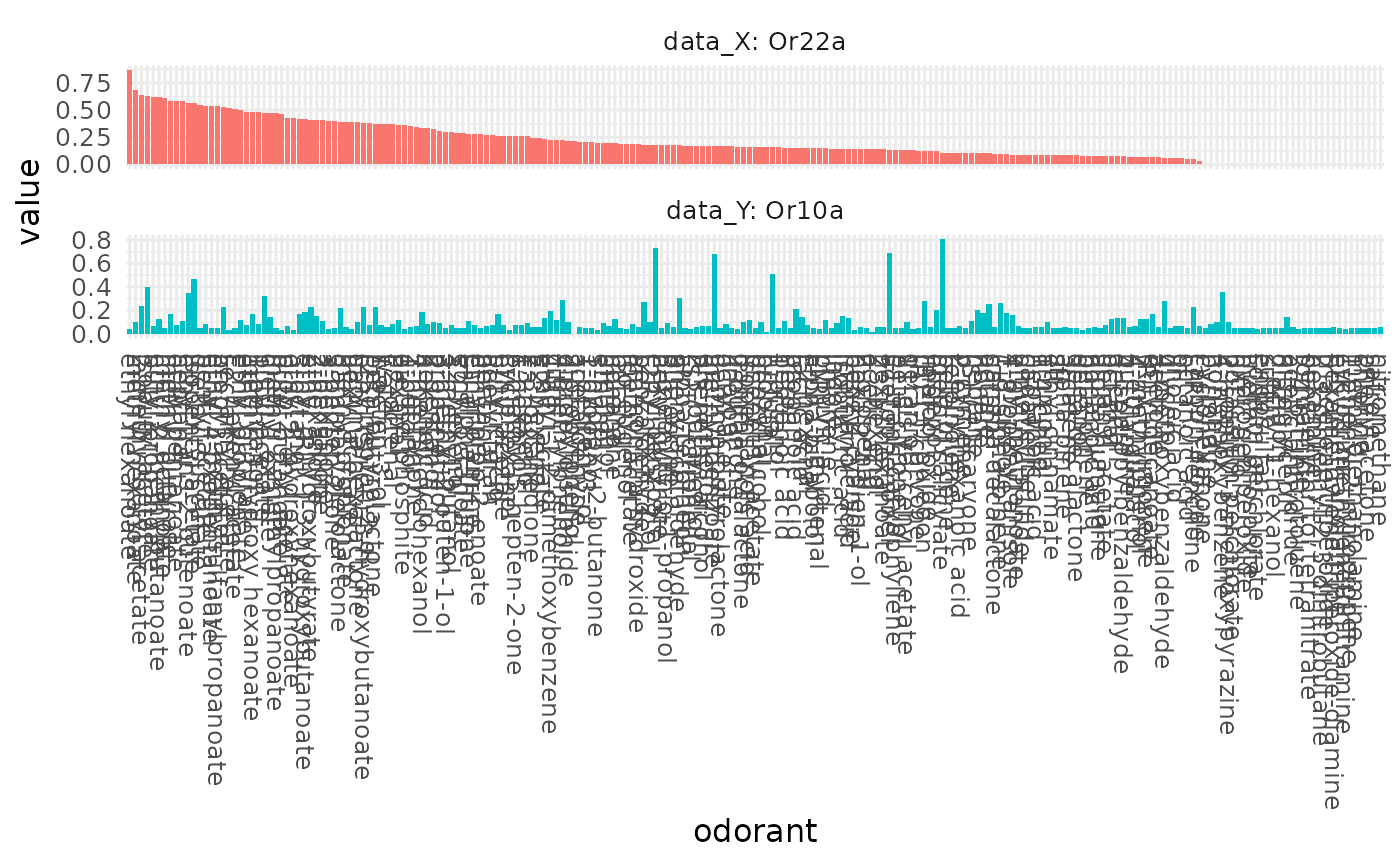Orderdered bar plots for two studies, allowing for an easy comparison of the two studies / response profiles'.

## Usage

``dplot_compare_profiles(x, y, by.x, by.y, tag = "Name", base_size = 12)``

## Arguments

x

the input data frame the first response profile will be taken from

y

the input data frame the second response profile will be taken from (x will be taken if y is missing)

by.x

character string, specifying a column in x

by.y

character string, specifying a column in y

tag

character, the chemical identifier that will be used as odorant label.

base_size

numeric, the base font size for the ggplot2 plot

## Author

Daniel Münch <daniel.muench@uni-konstanz.de>

## Examples

``````# load data
library(DoOR.data)
library(DoOR.functions)
data(Or22a)
data(door_response_range)
data(door_response_matrix)

# compare the Hallem and the Pelz data set for Or22a
dplot_compare_profiles(x = Or22a, y = Or22a,
by.x = "Hallem.2006.EN",
by.y = "Pelz.2006.AntEC50")# comparedata from two different sensory neurons and add odorant labels
dplot_compare_profiles(x = cbind(door_response_matrix, InChIKey =
rownames(door_response_matrix)), y = cbind(door_response_matrix, InChIKey =
rownames(door_response_matrix)), by.x = "Or22a", by.y = "Or10a")``````